International
Tables for
Crystallography
Volume C
Mathematical, physical and chemical tables
Edited by E. Prince

International Tables for Crystallography (2006). Vol. C, ch. 1.3, p. 14

Section 1.3.5. Calculation of the twin element

E. Kocha

aInstitut für Mineralogie, Petrologie und Kristallographie, Universität Marburg, Hans-Meerwein-Strasse, D-35032 Marburg, Germany

1.3.5. Calculation of the twin element

| top | pdf |

If the twin element cannot be recognized by direct macroscopic or microscopic inspection, it may be calculated as described below. Given are two analogous bases a, b, c and a′, b′, c′ referring to the two twin components. If possible, both basis systems should be chosen with the same handedness. If no such bases exist, the twin is a reflection twin and one of the bases has to be replaced by its centrosymmetrical one, e.g. a′, b′, c′ by −a′, −b′, −c′. The relation between the two bases is described by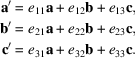The coefficients eij have to be obtained by measurement.

Basis a, b, c may be mapped onto a′, b′, c′ by a pure rotation that brings a to a′, b to b′, and c to c′. To derive the direction of the rotation axis, calculate the three vectors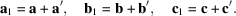a1, b1, c1 bisect the angles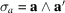,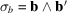, and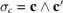, respectively. Calculate three further vectors of arbitrary length a2, b2, c2 which are perpendicular to the planes defined by a and a′, b and b′, and c and c′, respectively, from the scalar products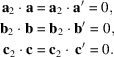The plane defined by a1 and a2 is perpendicular to the plane defined by a and a′ and bisects the angle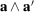. Analogous planes refer to b1 and b2, and c1 and c2. Vectors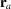,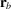, and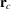lying within one of these planes may be described as linear combinations of a1 and a2, b1 and b2, or c1 and c2, respectively: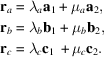The common intersection line of these three planes is parallel to the twin axis. It may be calculated by solving any of the three equations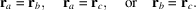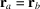: choose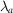arbitrarily equal to 1.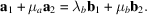Solve the inhomogeneous system of three equations that corresponds to this vector equation for the three variables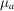,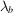, and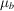. Calculate the vector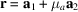. Its components with respect to a, b, c describe the direction of the twin axis.

The angle τ of the twin rotation may then be calculated by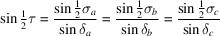with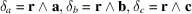.

If the basis a, b, c is orthogonal, τ may be obtained from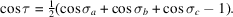If the coefficients of r are rational and τ equals 180°, then r describes the direction either of the twofold twin axis or of the normal of the twin plane. If r is rational and τ equals 60, 90 or 120°, r is parallel to the twin axis. If r is irrational, but τ equals 180° and there exists, in addition, a net plane perpendicular to r, this net plane describes the twin plane.

If none of these conditions is fulfilled, one has to repeat the calculations with a differently chosen basis system for one of the twin components. The number of possibilities for this choice depends on the lattice symmetry. The following list gives all equivalent basis systems for all descriptions of Bravais lattices used in IT A (2005):

 aP: a, b, c; mP, mS (unique axis b): a, b, c; −a, b, −c; mP, mS (unique axis c): a, b, c; −a, −b, c; oP, oS, oI, oF: a, b, c; −a, −b, c; −a, b, −c; a, −b, −c; tP, tI: a, b, c; −a, −b, c; −a, b, −c; a, −b, −c; b, −a, c; −b, a, c; b, a, −c; −b, −a, −c; hP: a, b, c; b, −a − b, c; −a − b, a, c; b, a, −c; −a − b, b, −c; a, −a − b, −c; −a, −b, c; −b, a + b, c; a + b, −a, c; −b, −a, −c; a + b, −b, −c; −a, a + b, −c; hR (hexagonal description): a, b, c; b, −a − b, c; −a − b, a, c; b, a, −c; −a − b, b, −c; a, −a − b, −c; hR (rhombohedral description): a, b, c; b, c, a; c, a, b; −b, −a, −c; −a, −c, −b; −c, −b, −a; cP, cI, cF: a, b, c; b, c, a; c, a, b; −a, −b, c;  −b, c, −a; c, −a, −b; −a, b, −c; b, −c, −a;  −c, −a, b; a, −b, −c; −b, −c, a; −c, a, −b;  −b, −a, −c; −a, −c, −b; −c, −b, −a; b, a, −c;  a, −c, b; −c, b, a; b, −a, c; −a, c, b; c, b, −a; −b, a, c; a, c, −b; c, −b, a.

References

International Tables for Crystallography (2005). Vol. A, Space-group symmetry, edited by Th. Hahn. Heidelberg: Springer.